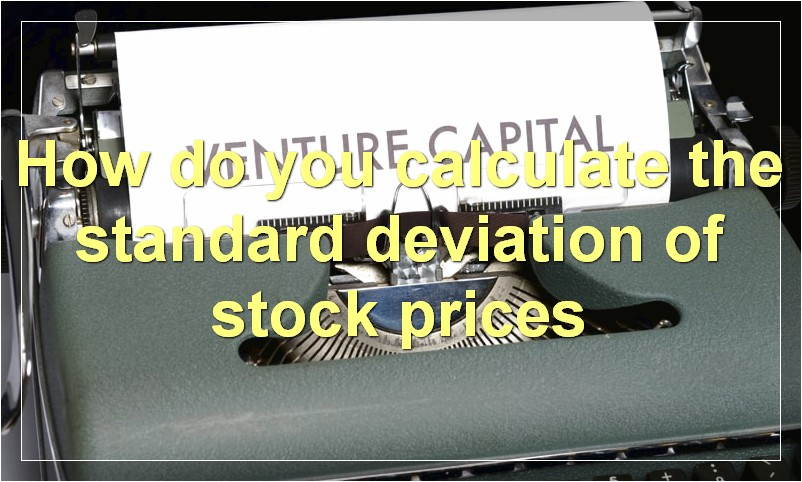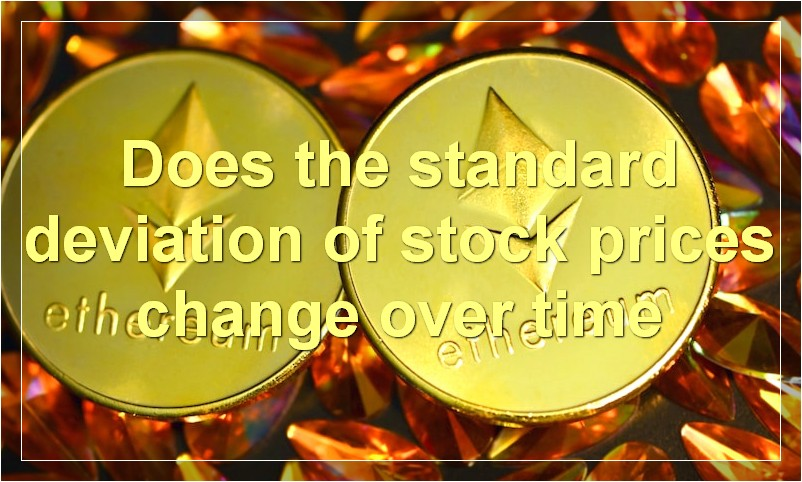# The Standard Deviation Of Stock Prices: Everything You Need To Know

The standard deviation is a key metric for determining the volatility of stock prices. By understanding how it works, you can make smarter investment decisions.

## What is the standard deviation of stock prices

When it comes to stocks, there are a lot of things that go into making sure that they are stable. This includes the standard deviation of stock prices. In order to ensure that your investment is secure, you need to understand what this term means and how it affects the prices of stocks.

The standard deviation of stock prices is a statistical measure that shows how much the prices of a particular stock differ from the average price. This number can be used to predict how likely it is for the stock to fluctuate in the future. A higher standard deviation means that the prices are more likely to vary greatly, while a lower number indicates that the prices are more stable.

This information is important because it can help you decide when to buy or sell a particular stock. If you know that the prices are going to be unstable, you may want to wait until they settle down before making any decisions. On the other hand, if you feel confident about the stability of the stock, you may want to buy sooner rather than later.

No matter what you decide to do, make sure that you do your research and understand the risks involved. Standard deviation is just one factor that you need to consider when making investment decisions.

## How do you calculate the standard deviation of stock pricesThe standard deviation of stock prices is a measure of how much the prices fluctuate. It is calculated by taking the square root of the variance, which is the average of the squared differences from the mean.

## What is a good standard deviation for stock prices

There is no definitive answer to this question as different investors will have different opinions on what is considered a good standard deviation for stock prices. Some may argue that a lower standard deviation indicates that a stock is less volatile and therefore less risky, while others may view a higher standard deviation as a sign that the stock has more potential for growth. Ultimately, it is up to the individual investor to decide what level of risk they are comfortable with and what they consider to be a good standard deviation for stock prices.

## How does the standard deviation of stock prices affect investors

The standard deviation is a statistical measure of dispersion, calculated as the square root of the variance. It measures how much variation or “dispersion” there is from the average (mean) stock price. A higher standard deviation means that prices are more spread out from the mean and thus tend to be more volatile. A lower standard deviation means that prices are clustered more closely around the mean and thus tend to be less volatile.

For investors, standard deviation is a important metric because it can give them a sense of how volatile a particular stock is. If a stock has a high standard deviation, it means that its price is more likely to fluctuate sharply in either direction (up or down). This can make it riskier to invest in, but also potentially more profitable if the price does indeed go up. Conversely, a stock with a low standard deviation is less likely to see sharp price movements, making it a safer investment but also one with less potential for gain.

## What are the benefits of having a low standard deviation for stock prices

A low standard deviation for stock prices indicates that the prices are not volatile and tend to remain stable. This is considered to be a good thing by investors because it means that their investment is less likely to lose value suddenly. Additionally, a low standard deviation makes it easier to predict future price movements, which can give investors an edge in making profitable trades.

## Does the standard deviation of stock prices change over timeThere is no simple answer to this question as the standard deviation of stock prices can be affected by a number of different factors. However, it is generally accepted that the standard deviation of stock prices tends to increase over time. This is due to the fact that stock prices are usually more volatile in the long-term than in the short-term. As such, investors should be aware that the standard deviation of stock prices can change over time and they should be prepared for periods of increased volatility.

## What factors affect the standard deviation of stock prices

There are a number of factors that can affect the standard deviation of stock prices. One is the overall market volatility. When the market is volatile, stock prices tend to move up and down more rapidly, which can increase the standard deviation. Another factor is the company’s financial stability. If a company is in good financial health, its stock price is likely to be more stable than a company with financial difficulties. Finally, stock prices can be affected by news events. If there is positive news about a company, its stock price is likely to go up. Similarly, if there is negative news, the stock price is likely to go down.

## Is there a relationship between risk and the standard deviation of stock prices

There is a well-known relationship between risk and return in the investment world. Higher returns are generally associated with higher risks. The standard deviation of stock prices is a measure of risk. It is a statistical measure of how much the prices of a stock fluctuate over time. A higher standard deviation means that the prices of the stock are more volatile and therefore riskier.

So, there is definitely a relationship between risk and the standard deviation of stock prices. However, it is important to remember that this is not a perfect relationship. There are many other factors that affect risk and return, such as the type of asset, the geographical location, the political environment, etc.

## What is the historical standard deviation of stock prices

A historical standard deviation is a statistical measure of how much past stock prices have fluctuated. It’s calculated using data from past years, and it can be used to predict how stocks might move in the future.

Why is the historical standard deviation important?

The historical standard deviation can be a helpful tool for investors. It can help them understand how volatile (or risky) a stock has been in the past, which can give them a better idea of what to expect in the future.

What does a high historical standard deviation mean?

A high historical standard deviation means that a stock’s price has been very volatile in the past. This could mean that the stock is riskier than other stocks, and that its price could go up or down a lot in the future.

What does a low historical standard deviation mean?

A low historical standard deviation means that a stock’s price hasn’t fluctuated much in the past. This could mean that the stock is less risky than other stocks, and that its price is less likely to change dramatically in the future.

## What is the expected future standard deviation of stock prices

It is difficult to predict the future standard deviation of stock prices with any degree of accuracy. However, if we look at the past volatility of stock prices, we can get a general idea of what to expect in the future. Based on historical data, it is reasonable to expect that the future standard deviation of stock prices will be around 10%.